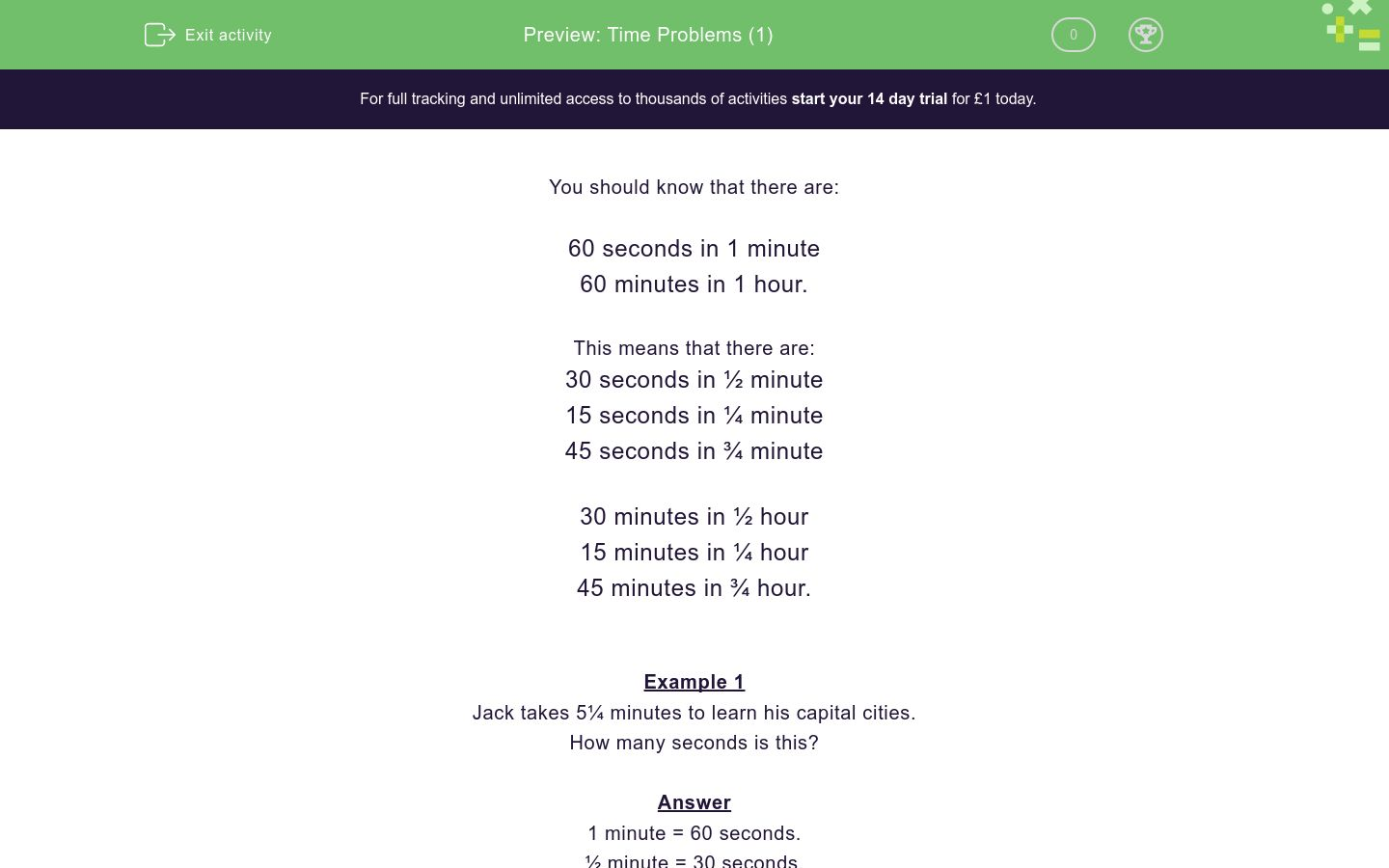# Time Problems (1)

In this worksheet, students must know how many seconds, minutes and hours there are in various time periods, including halves and quarters.Key stage:  KS 2

Curriculum topic:   Measurement

Curriculum subtopic:   Solve Time Problems

Difficulty level:#### Worksheet Overview

You should know that there are:

60 seconds in 1 minute

60 minutes in 1 hour.

This means that there are:

30 seconds in ½ minute

15 seconds in ¼ minute

45 seconds in ¾ minute

30 minutes in ½ hour

15 minutes in ¼ hour

45 minutes in ¾ hour.

Example 1

Jack takes 5¼ minutes to learn his capital cities.

How many seconds is this?

Answer

1 minute = 60 seconds.

½ minute = 30 seconds.

¼ minute = 15 seconds.

5¼ minutes = 5 × 60 + 15 = 300 + 15 = 315 seconds.

Example 2

Ahmed starts work at 9 a.m. and finishes at 4:30 p.m.

For how many minutes is he at work?

Answer

From 9 a.m. to 4 p.m. is 7 hours so Ahmed is at work for 7½ hours.

1 hour = 60 minutes.

½ hour = 30 minutes.

7½ hours = 7 × 60 + 30 = 420 + 30 = 450 minutes.

### What is EdPlace?

We're your National Curriculum aligned online education content provider helping each child succeed in English, maths and science from year 1 to GCSE. With an EdPlace account you’ll be able to track and measure progress, helping each child achieve their best. We build confidence and attainment by personalising each child’s learning at a level that suits them.

Get startedTry an activity or get started for free